Back to Kemp Acoustics HomeNext: Results Up: Multimodal radiation impedance of Previous: Multimodal radiation impedance of   Contents

## Analysis

Expressing (3.13) in cylindrical coordinates for a cylindrical duct of radius
:(3.14)

where(3.15)

As discussed in section 2.4.1, we will be treating cylindrically symmetric modes only. From equation (2.48), the mode profile on the surface is(3.16)

whereis theth zero of the Bessel function
and is tabulated in appendix A.

Now we will givein terms of
, a dummy variable of integration. We will show that all the other variables of integration will then have an analytic solution. Sonine's integral from Watson  p.416 gives:
(3.17)

The integrand is imaginary when
and real when
. Care must be taken when choosing the sign of
with the negative and imaginary interpretation taken here when. Notice that we have been using the opposite sign convention from Zorumski  for the imaginary part throughout because we are assuming a time factor of
rather than.

Neumann's addition formula  p.358 is
(3.18)

which can be substituted into (3.17) to give
(3.19)

Now substituting (3.19) into (3.14) we get
(3.20)

Note that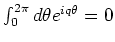unless, in which case it is equal to. Integrating byandthen gives a factor of. When rearranged, the integral can be reduced to(3.21)

where
(3.22)

The integration in equation (3.22) can be found analytically (see equation (A.1) in appendix A):(3.23)

The four dimensional integral has now been reduced to a one dimensional integration, with the variable
(and therefore the singularity mentioned at the end of section 3.3.1) eliminated. Noticing that in equation (3.21) the integral is real for
and imaginary for
, we split the integral into real and imaginary parts with variables
and
respectively.
(3.24)

Nowand
. Remembering that the negative imaginary interpretation should be taken for the resulting
we get
(3.25)

The first integral can be performed by numerical integration using Simpson's rule or an equivalent. In the second integral, however, the range extends to infinity. The integrand is an oscillatory function ofwhose amplitude of oscillation decays exponentially to
typically at around
. Numerical integration can then be performed from 0 and 10 without incurring any significant numerical errors.

Back to Kemp Acoustics HomeNext: Results Up: Multimodal radiation impedance of Previous: Multimodal radiation impedance of   Contents
Jonathan Kemp 2003-03-24